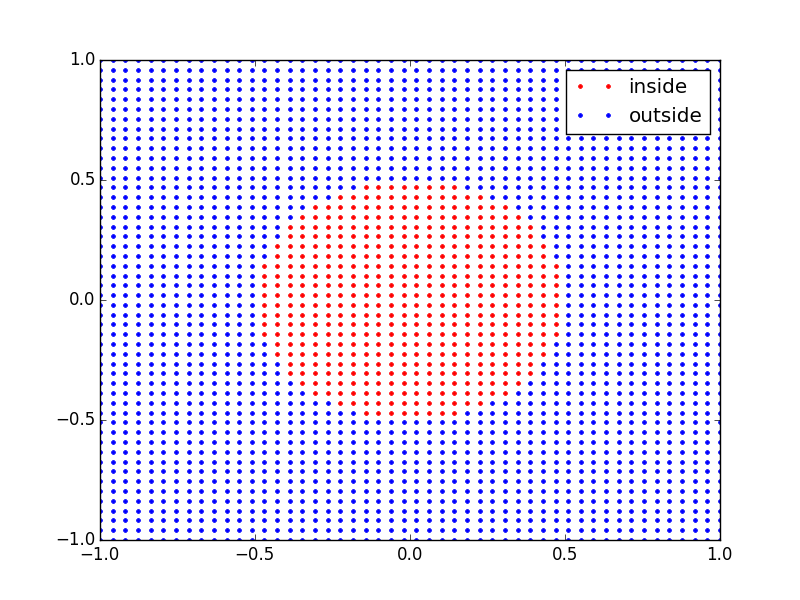# Point Inside A Material¶

This demo shows how the world.contains() method can be used to query the world for all primitives that intersect the test point. This simple scene contains a Sphere at the origin of radius 0.5m. A grid of test points is generated in the x-y plane. Each point is tested to see if it lies inside the sphere. The test points are plotted in the figure, coloured by their true (inside)/false (outside) categorisation.


# External imports
import numpy as np
import matplotlib.pyplot as plt

# Internal imports
from raysect.optical import World, translate, Point3D
from raysect.optical.library import schott
from raysect.primitive import Sphere

"""
World contains point
--------------------

This demo shows how the world.contains() method can be used to query the world for
all primitives that intersect the test point. This simple scene contains a Sphere
at the origin of radius 0.5m. A grid of test points is generated in the x-y plane.
Each point is tested to see if it lies inside the sphere.
"""

world = World()

# Place test sphere at origin
sphere = Sphere(radius=0.5, transform=translate(0, 0, 0), material=schott("N-BK7"))
sphere.parent = world

# Construct test points in x-y plane

xpts = np.linspace(-1.0, 1.0)
ypts = np.linspace(-1.0, 1.0)

x_inside = []
y_inside = []
x_outside = []
y_outside = []

for x in xpts:
for y in ypts:

test_point = Point3D(x, y, 0)

# For each test point, call world.contains() which returns a list of primitives that contain the test point.
primitives = world.contains(test_point)

# Next we see if the sphere is in the list of primitives returned. If yes, the test point lies inside the
# sphere, otherwise it must be outside.
if sphere in primitives:
x_inside.append(x)
y_inside.append(y)
else:
x_outside.append(x)
y_outside.append(y)

plt.figure()
plt.plot(x_inside, y_inside, '.r', label='inside')
plt.plot(x_outside, y_outside, '.b', label='outside')
plt.legend()
plt.show()